## MINI CLUBMAN Hampton限量版车型小图解

2014年10月30日 09:18 来源：www.chextx.com 超过：次关注 阅读全文

在“MINI”品牌50岁生日之际，MINI发布了两款别具特色的车型：MINI 50 Mayfair和MINI 50 Camden。我的同事李店斌在《主打配置MINICooper50周年纪念版实拍》一文中对于MINI 50 Mayfair限量版车型丰富的配置印象深刻。6月24日，MINI厂方将推出第三款50周年限量版车型——MINI CLUBMAN Hampton，这款特别版车型是为了庆祝CLUBMAN/Traveller车型50周年而推出的。除了延续MINI限量版车型的高配置以外，这款车型还配置了中国“米粉”颇为关注的MINI Visual Boost 多媒体系统。

这款特别版的MINI车型外观主要是在车身颜色、头灯、标识、轮毂样式等几处发生变化，这一点，MINI CLUBMAN Hampton同之前两款50周年纪念版车型类似。同时，这款车也与 MINI Mayfair和MINI Camden一样，限量生产一年。凭借这一点就会点燃起许多“米粉”的购买欲望。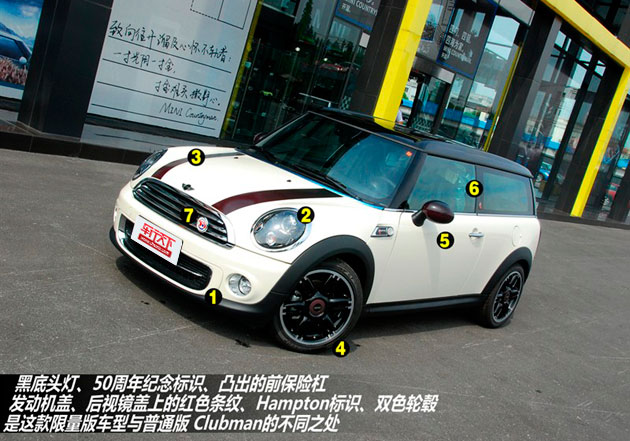实拍的MINI CLUBMAN Hampton 使用胡椒白的车身颜色涂装，黑色平直的车顶与车身颜色形成对比，搭配红色波纹点缀的发动机盖和后视镜，看起来很个性！这款限量版车型颜色除了胡椒白可以选择之外，还提供了礁蓝色、午夜黑以及日食灰供年轻消费者选择。不过，专为这款车型打造的礁蓝色涂装车型并未在经销商处看到。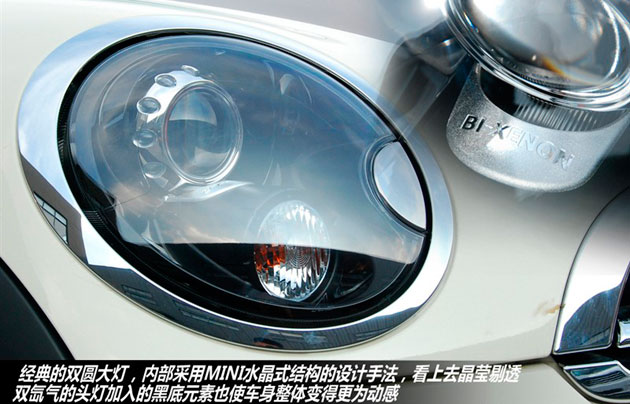如果不仔细看几乎看不出它和普通版MINI CLUBMAN有什么区别。除了中网格栅处增添的50周年纪念徽章以外，双氙气的头灯也入黑底元素，前保险杠进气格栅较普通版 MINI CLUBMAN车型略微凸出，这两处改变对整车的运动气息有着不小的提升。需要注意的是，这款双氙气头灯将以选装包的形式出现。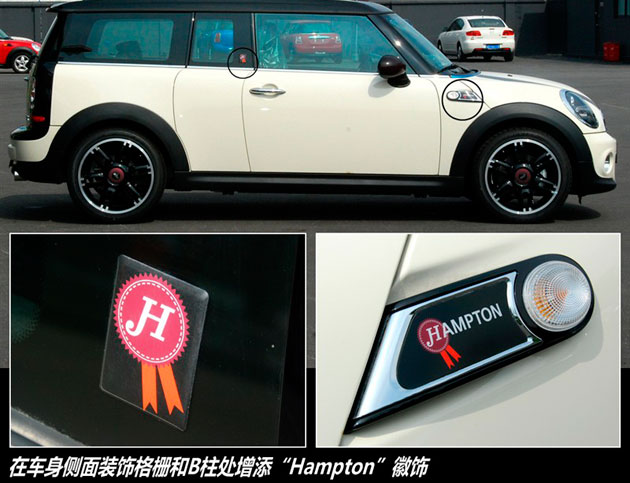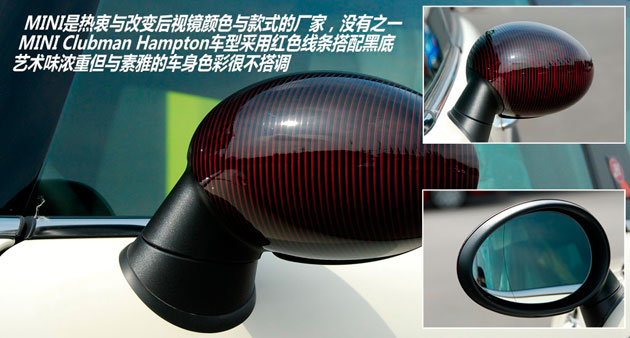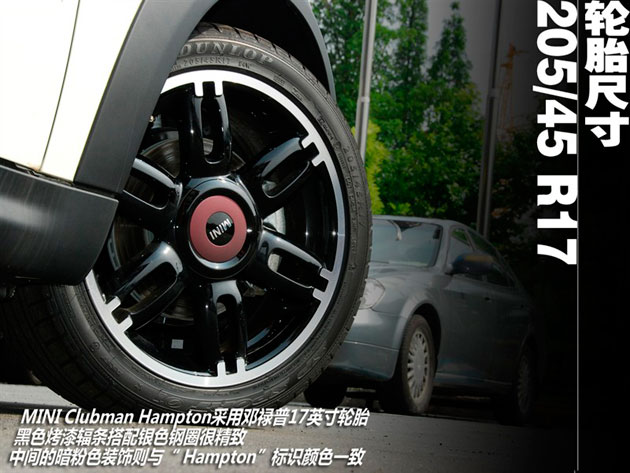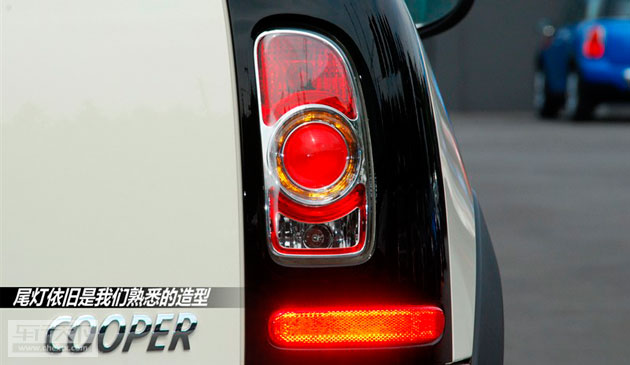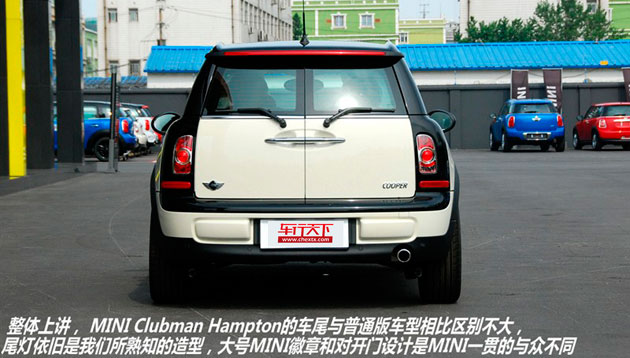除了时尚的车身颜色可供选择外，在车身侧面装饰格栅、B柱处增添“Hampton”徽饰也是与普通版最直接的不同。要想凸显出限量身份的不同，更改轮毂是最直接的办法之一，为了提升运动效果MINI CLUBMAN Hampton将轮胎尺寸升级到17英寸，轮胎的尺寸为205/45 R17，双色的设计也与车身颜色很搭调。这种样式的轮毂只是MINI限量版车型多种轮毂选装件其中的一款。对于MINI的车主来说，购买MINI时一定要考虑好车身的颜色搭配哪款轮毂会更适合自己。

1 2

﻿
• 快速找车
• 选择品牌
• 选择品牌
• A  奥迪
• A  阿斯顿·马丁
• A  阿尔法·罗密欧
• B  宝沃
• B  布加迪
• B  巴博斯
• B  保时捷
• B  宾利
• B  奔驰
• B  宝马
• B  本田
• B  别克
• B  标致
• B  比亚迪
• B  宝骏
• B  北汽制造
• B  北汽新能源
• B  北汽幻速
• B  北汽威旺
• B  北京汽车
• B  奔腾
• B  北汽绅宝
• B  北汽昌河
• C  长安欧尚
• C  长安
• C  长安凯程
• C  长城
• D  大众
• D  道奇
• D  DS
• D  东南
• D  东风风神
• D  东风风行
• D  东风小康
• D  东风风度
• D  东风
• F  福特
• F  丰田
• F  菲亚特
• F  法拉利
• F  福田
• F  福迪
• F  福汽启腾
• G  观致
• G  广汽传祺
• G  广汽吉奥
• G  GMC
• H  红旗
• H  汉腾汽车
• H  哈弗
• H  哈飞
• H  海格
• H  海马
• H  华颂
• H  黄海
• H  华泰
• H  恒天
• J  几何汽车
• J  捷达
• J  吉利汽车
• J  捷豹
• J  Jeep
• J  江淮
• J  江铃
• J  金杯
• J  九龙
• J  金旅
• K  凯翼
• K  凯迪拉克
• K  克莱斯勒
• K  科尼塞克
• K  卡威
• K  开瑞
• L  路虎
• L  林肯
• L  劳斯莱斯
• L  兰博基尼
• L  雷克萨斯
• L  铃木
• L  领克
• L  雷诺
• L  理念
• L  力帆
• L  莲花汽车
• L  猎豹
• L  路特斯
• L  陆风
• M  马自达
• M  MG
• M  MINI
• M  玛莎拉蒂
• M  摩根
• M  迈凯轮
• N  纳智捷
• O  欧拉
• O  欧宝
• O  讴歌
• O  欧朗
• Q  奇瑞
• Q  起亚
• Q  启辰
• R  日产
• R  荣威
• R  瑞麒
• S  SERES赛力斯
• S  三菱
• S  斯威汽车
• S  萨博
• S  smart
• S  斯柯达
• S  斯巴鲁
• S  思铭
• S  双龙
• S  上汽大通
• S  双环
• T  特斯拉
• T  腾势
• W  蔚来
• W  沃尔沃
• W  WEY
• W  五菱汽车
• W  五十铃
• W  威兹曼
• W  威麟
• X  现代
• X  雪佛兰
• X  星途
• X  雪铁龙
• X  小鹏汽车
• X  西雅特
• Y  一汽
• Y  英菲尼迪
• Y  英致
• Y  依维柯
• Y  野马汽车
• Y  永源
• Z  众泰
• Z  中华
• Z  中兴
• Z  知豆
• 选择车系
• 选择车系
• 车型对比
• 选择品牌
• 选择品牌
• A  奥迪
• A  阿斯顿·马丁
• A  阿尔法·罗密欧
• B  宝沃
• B  布加迪
• B  巴博斯
• B  保时捷
• B  宾利
• B  奔驰
• B  宝马
• B  本田
• B  别克
• B  标致
• B  比亚迪
• B  宝骏
• B  北汽制造
• B  北汽新能源
• B  北汽幻速
• B  北汽威旺
• B  北京汽车
• B  奔腾
• B  北汽绅宝
• B  北汽昌河
• C  长安欧尚
• C  长安
• C  长安凯程
• C  长城
• D  大众
• D  道奇
• D  DS
• D  东南
• D  东风风神
• D  东风风行
• D  东风小康
• D  东风风度
• D  东风
• F  福特
• F  丰田
• F  菲亚特
• F  法拉利
• F  福田
• F  福迪
• F  福汽启腾
• G  观致
• G  广汽传祺
• G  广汽吉奥
• G  GMC
• H  红旗
• H  汉腾汽车
• H  哈弗
• H  哈飞
• H  海格
• H  海马
• H  华颂
• H  黄海
• H  华泰
• H  恒天
• J  几何汽车
• J  捷达
• J  吉利汽车
• J  捷豹
• J  Jeep
• J  江淮
• J  江铃
• J  金杯
• J  九龙
• J  金旅
• K  凯翼
• K  凯迪拉克
• K  克莱斯勒
• K  科尼塞克
• K  卡威
• K  开瑞
• L  路虎
• L  林肯
• L  劳斯莱斯
• L  兰博基尼
• L  雷克萨斯
• L  铃木
• L  领克
• L  雷诺
• L  理念
• L  力帆
• L  莲花汽车
• L  猎豹
• L  路特斯
• L  陆风
• M  马自达
• M  MG
• M  MINI
• M  玛莎拉蒂
• M  摩根
• M  迈凯轮
• N  纳智捷
• O  欧拉
• O  欧宝
• O  讴歌
• O  欧朗
• Q  奇瑞
• Q  起亚
• Q  启辰
• R  日产
• R  荣威
• R  瑞麒
• S  SERES赛力斯
• S  三菱
• S  斯威汽车
• S  萨博
• S  smart
• S  斯柯达
• S  斯巴鲁
• S  思铭
• S  双龙
• S  上汽大通
• S  双环
• T  特斯拉
• T  腾势
• W  蔚来
• W  沃尔沃
• W  WEY
• W  五菱汽车
• W  五十铃
• W  威兹曼
• W  威麟
• X  现代
• X  雪佛兰
• X  星途
• X  雪铁龙
• X  小鹏汽车
• X  西雅特
• Y  一汽
• Y  英菲尼迪
• Y  英致
• Y  依维柯
• Y  野马汽车
• Y  永源
• Z  众泰
• Z  中华
• Z  中兴
• Z  知豆
• 选择车系
• 选择车系
• 选择车型
• 选择车型
• 意见反馈